Home » Build your First Linear Regression Model in Qlik Sense

# Build your First Linear Regression Model in Qlik Sense

## Overview

• Qlik is widely associated with powerful dashboards and business intelligence reports
• Did you know that you can use the power of Qlik to perform predictive modeling and build models?
• Learn how to do just that with this really cool guide on building a linear regression model using Qlik Sense

## Introduction

“Can I use Qlik Sense to create a WHAT-IF analysis by building a simple linear regression model so that my business users can forecast future profits based on the target sales?”

An intriguing question! Qlik is widely associated with building dashboards and business intelligence reports, not predictive modeling. If you were of the same thought – you’re not alone!

Qlik is like a wind in the back for any business leader. It makes analyzing and presenting data to end-users extremely easy and fast. No wonder Qlik is regularly named as a Leader in Gartner’s Magic Quadrant for business intelligence and analytics platforms.What I really about Qlik is that its associative model offers free form data discovery. This helps our end user quickly find trends and outliers to gain meaningful insights. Qlik is well known for its associative model and for blazing speed with which it reveals associations among fields within a data model.

With its paradigm shift of showing all data, including outliers, our clients and stakeholders can quickly find insights to make critical business decisions. The applications of Qlik spans across multiple industries like:

• In healthcare, an insurance company may want to predict the future cost of patient care using prior costs, demographics, and diagnosis
• Product defects can be predicted using process efficiency based on prior defects and equipment accuracy in the manufacturing sector
• In human resources, we can predict the future payroll cost of an employee based on his or her age and experience

Think of the possibilities – they are endless!

After reading this article, you will be able to wear a data scientist’s hat as both QlikView and Qlik Sense offer a plethora of statistical functions that you can leverage to build your first predictive model using linear regression. So let’s begin!

1. Introduction to Simple Linear Regression
2. Implementing Linear Regression in Qlik
3. Comparing our Results with a Model Built using Python

## Introduction to Simple Linear Regression

Let’s begin with the concept of regression analysis. It is a form of predictive modeling that reveals the relationship between an independent and a dependent variable. This is perhaps the most common technique aspiring data science professionals learn first.

Regression is used to assess the contribution of one or more “causing” variables (independent variables) to one “caused” (dependent) variable. We can also use it to predict the value of the dependent variable from the values of independent variables. Some popular examples include predicting the price of a house, the salary of an employee, and so on (I’m sure your mind must be swirling with ideas!).

When there is only one independent variable and when the relationship can be expressed as a straight line, the procedure is called simple linear regression.

A straight line can be defined by the mathematical equation y = mx + b: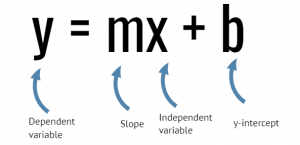• y is a dependent variable and represented on the vertical axis
• x is an independent variable and represented on the horizontal axis
• m is the slope (amount of change in y corresponding to one-unit increase in x)
• b is the intercept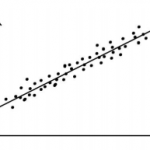Source: http://www.statstutor.ac.uk

The regression procedure fits the best possible straight line to an array of data points. If no single line can be drawn such that all the points fall on it, what is the “best” line? Think about it before you read the answer.

The best line is the one that minimizes the distance of all the data points to the line.

The correlation coefficient indicates the strength of the relationship between the independent and the dependent variable whereas the coefficient of determination (r-squared) explains to what extent the variance of the independent variable explains the variance of the dependent variable.

A correlation coefficient close to 1 indicates a positive relationship between the independent and the dependent variable and a coefficient of determination closer to 1 indicates a good fit of data to the predictive model.

Armed with this knowledge, we can create our first simple linear regression model in either Qlik Sense or QlikView.

## Implementing Linear Regression in Qlik

Recently, I stumbled upon this very interesting article that shows the nexus between teen pregnancy and poverty rate in America. These facts are worth pondering about the reasons why teen pregnancy leads to a higher poverty rate:

• Only 38 percent of girls who have a child before the age of 18 get their high school diplomas by 22
• Two-thirds of teen mothers who move out of their family home live in poverty, and a similar share receive public benefits in the first year of their child’s life
• Seventy-eight percent of children born to teenage mothers who never married and who did not graduate from high school live below the federal poverty level

It’s a problem that we all should be aware of and if we can help in any way, we should at least try. I was lucky enough to find a dataset around this on Pennsylvania State University’s statistics website, STAT462.

So, we’ll be using this dataset to create a simple linear regression model in Qlik Sense. Go ahead and save it to your machine. Here is a snapshot of the dataset: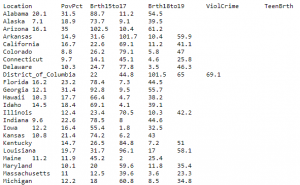This dataset of size n = 51 is for the 50 states and the District of Columbia in the United States.

So, let’s look at the steps and I want you to follow along in Qlik Sense as we go through them.

### Step 1: Create a Scatterplot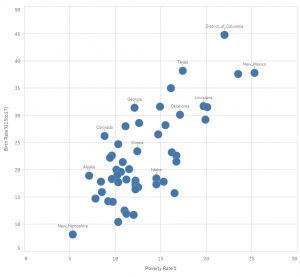### Step 2: Calculate correlation coefficient

Create a text and image chart using the below expression: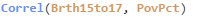### Step 3: Calculate the coefficient of determination

Create a text and image chart using this expression: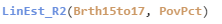### Step 4: Calculate the slope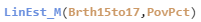### Step 5: Calculate the y-intercept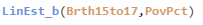### Step 6: Create a variable x with initial value = 0

This variable will allow us to change the independent variable value, birth rate (15 to 17), to predict the poverty rate. Click on the variable option from the bottom left corner of the sheet editor: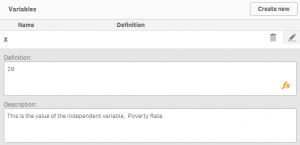### Step 7: Calculate the predicted Birth Rate for teenage group (15 to 17)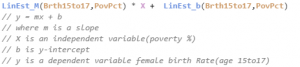As stated here,  our Qlik Sense Linear Regression model matches the fitted line equation:

Y = 1.373X + 4.267

At 0% poverty rate, teenage birth rate would be 4.27%. A one-unit change in the value of the independent variable equates to 1.373 change in the value of the dependent variable.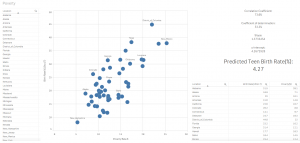What would be the teenage birth rate if the poverty rate is 15%? Here’s the answer: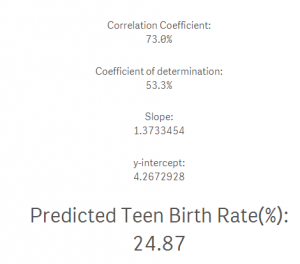I can now combine the power of associative engine to narrow down the list of States to predict the birth rate for a female age group 15 to 17 based on my selections using the poverty rate of 15%: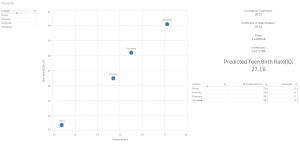Fabulous! Don’t you love the power of Qlik?

## Comparing our Results with a Model Built using Python

Next, we will build a similar simple regression model in Python using the Pandas and scikit-learn libraries. I want to compare the accuracy of the predictive model we created in Qlik Sense against one we will create in Python.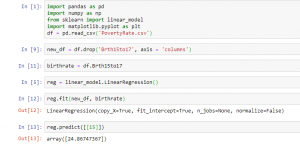The output from our Python simple regression model matches the one in Qlik Sense. Let’s compare the predictor value from our Qlik Sense linear regression model against the one we created in Python: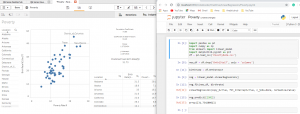## End Notes

We can create a simple regression model to show the “What-IF” scenario in Qlik Sense as long as we first validate that the relationship between the independent and the dependent variable is either positive or negative using a built-in correlation function to view relationship.

Besides, ensure that data is fit for modeling using the coefficient of determination (R-squared). If a value is closer to 1, then our data is suitable for simple regression modeling in Qlik Sense.

Shilpan Patel – Co-Founder, Analyticshub.io & Qlik Luminary 2018, 2019Shilpan is a Qlik Luminary and he is passionate about enabling students to realize their full potential through lifelong learning and mentorship. He believes that the best way to learn and master a skill is by doing. He has over 15 years of experience in data and analytics, and he has taught and mentored thousands of students.

You can also read this article on our Mobile APP•Alastair McIntosh says:
•Yogi Achilleos says: